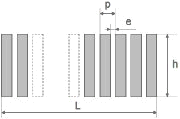# Design notes for phased array

## Grating lobes

When using a phased array transducer, delay laws are applied to each channel to generate a beam with a given refraction angle and focal distance. The ultrasonic beam is generated by the constructive interference of each transducer element’s contribution in the desired direction.

See phased array principle here >>

In some cases, this interference can also be constructive in other directions. These lobes of energy emitted outside the electronically driven direction are called grating lobes.
These energy lobes can interact with the part to be inspected in the same way as the main beam, and thus generate echoes causing interference to the inspection. Therefore they have to be avoided as much as possible.

The angle of the position of grating lobes in relation to the main beam is given by the following formula## sin θk = k . λ/ p – sinθ

θ = refracted angle of the main beam
θk = refracted angle of the grating lobe k (k: integer)
P = inter-element pitch (p) of the linear transducer
λ = wavelength in the medium under consideration

Note: this formula is only valid in the case of electronic deflection (linear delay law) . In case of electronic focusing, the angular  deviation between the main beam and the grating lobes is reduced.

#### Example for 70°SW in steel

From the above formula, the following general rule can be obtained :

• If p < λ/2, then no grating lobe is generated whatever the angle of the main beam.
• If p > λ, then there is always at least one grating lobe generated whatever the angle of the main beam.
• Between these two values, the grating lobes appear progressively according to the angle of the main beam.

The maximum pitch to avoid grating lobe is given by the formula:

## p < λ / (1 + sin θ)

In practice, for typical use in the 30-70° SW or LW range with a wedge for a 45° angle, a pitch of 1.0 λ gives good results.

## Lateral resolution along the plane of incidence

The following formula allows a good approximation to be made of the lateral resolution, and allows the correlation between the active aperture and the lateral resolution to be rapidly checked## W = 0.44.λ/ sin ( α / 2)

W= focal spot or beam width (at –6dB in emission reception)
λ = wave length in the medium under consideration
α = angle beneath which the active area is seen from the focal point

Note: This formula is a rough estimate when the hypothesis of electronic focusing at the focal point under consideration is made. It does not take into account the energy refraction/reflection law depending on the angle.

## Number of elements

Knowing the inter-element spacing required to avoid grating lobes, and the width of the active aperture, the number of elements necessary can be rapidly deduced.
If the appropriate number of electronic channels is not available for technical or economic reasons, the best possible compromise must be found, by readjusting the inter-element spacing, the frequency, and/or the active aperture (and thus the lateral resolution)

Note: Some linear array sensors are also used for electronic scanning. In this case, the beam is generated by only some of the elements. To calculate the lateral resolution, only the size of the active area is to be taken into account.